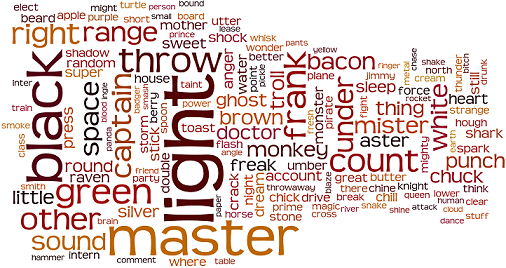Computer Science

# Sorting Algorithms: Level 2 challenges

Given a list of $n$ strings each of length $n$. What will be the worst case running time of sorting the strings in lexicographic order using merge-sort algorithm?Which sorting technique is the best for a list which is mostly sorted?Which type of sorting technique is best for sorting a linked list?

Do you know why?

×